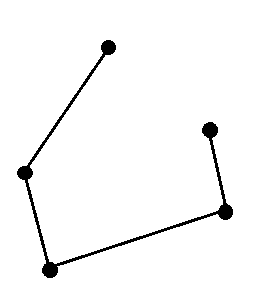# How to create a Hasse Diagram

Hasse Diagram is created for POSET or Partially Ordered Set. It means that there is a set of elements in which certain element are ordered, sequenced or arranged in some way. It is usually denoted as ≤, this is not “Less than, Equal to”, this symbol shows that elements are ordered.

Now, there is a relation among all the elements in the partial order set and it is some binary relation R, this relation must satisfy following properties

1. Reflexive
2. Anti-Symmetric
3. Transitive

### Example Problem

Suppose (z, |) is a partial order set where | is division operator and a | b means “a divides b”.

#### Reflexive Property

a | a => a divides itself and this is correct with respect to division. A number can divide itself.

#### Anti-Symmetric Property

a | b = b | a is correct and works only when a = b. If not, then it is not anti-symmetric.

#### Transitive Property

a | b => a divides b means b = a * m where m is some number. (1)
b | c => b divides c means c = b * n  where n is some number.  (2)
Using (1) and (2) we get
c = (a * m) * n
c = a * (m * n) which is same as (1). This proves the transitivity property.

### Hasse Diagram

Now that we know partial order set means and a Hasse Diagram is graphical representation of posets.

#### Rules for Hasse Diagram

1. If x < y, then in the graph x appears lower to y.
2. We draw line segment between x and y only if x cover y or y cover x, it means some order is maintained between them.
Consider an example
Let A be a set, A = { 1,  3,  5,  12,  15 }, relation between elements are a | b that is., a divides b.

#### Step 1

Due to the reflexive property all elements have direct edge to itself.

In this diagram, it shows the relations removed all the self-directing loops.

#### Step 2

The green lines shows transitivity, so we remove all the transitive lines. Out diagram look like the following.

#### Step 3

Replace all the vertices with dots and directed edges with ordinary lines. This final diagram is called the Hasse Diagram of poset.Hasse Diagram for A = { 1, 3, 5, 12, 15 } and relation a | b i.e., a divides b

### Exercise

Here is an exercise for you to practice. The prerequisite for Hasse Diagram is to know how to represent relations using graphs.

Let A be a poset, A = { 2,  4,  6,  8 } and the relation a | b is ‘a divides b. Draw a Hasse Diagram for the poset showing all the relations.